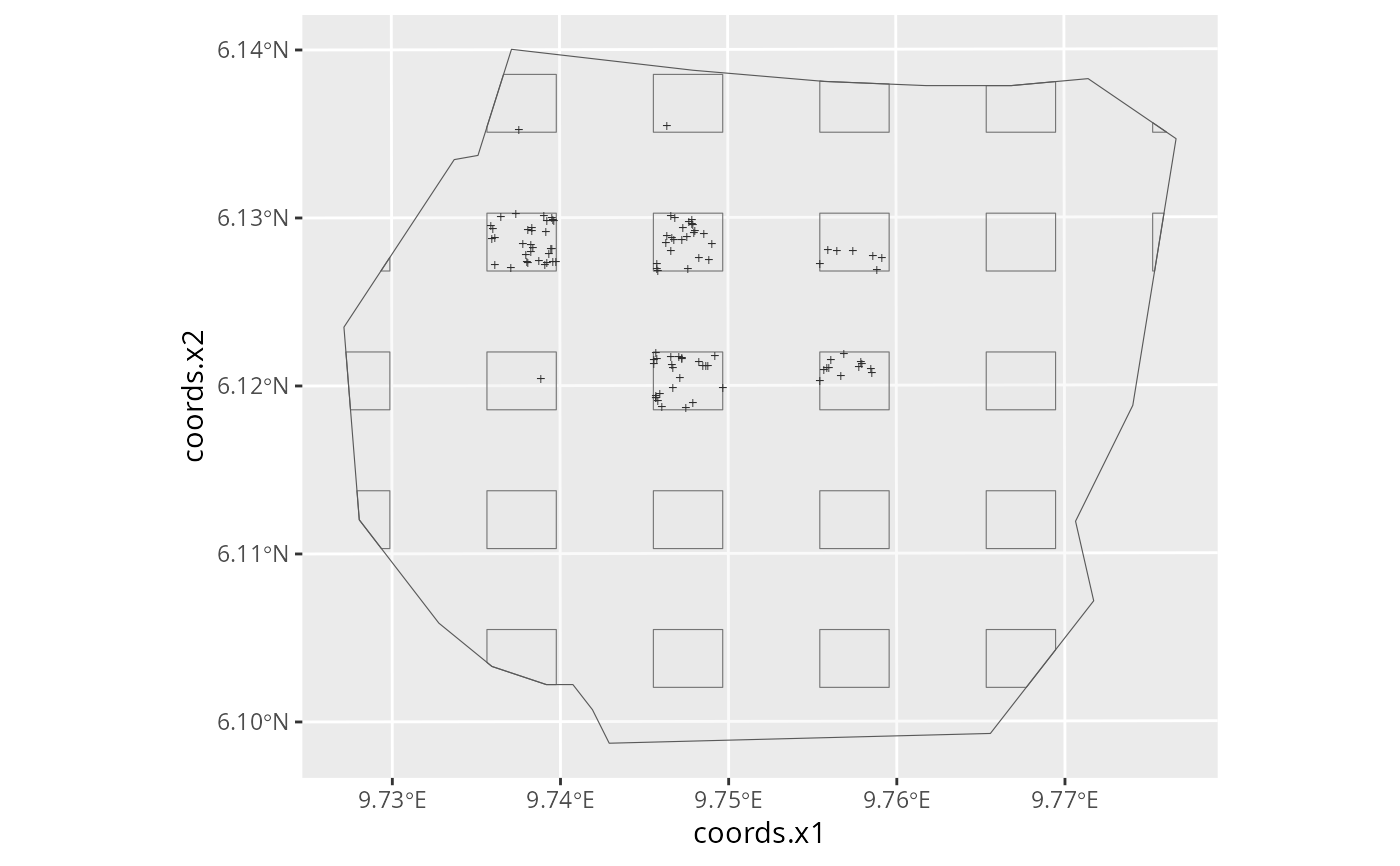Creates a plot sample on a regular grid with a random start location.

## Usage

plotsample(spdf, boundary, x.ppn = 0.25, y.ppn = 0.25, nx = 5, ny = 5)

## Arguments

spdf

A SpatialPointsDataFrame defining the points that are to be sampled by the plot sample.

boundary

A SpatialPolygonsDataFrame defining the survey boundary within which the points occur.

x.ppn

The proportion of the x=axis that is to be included in the plots.

y.ppn

The proportion of the y=axis that is to be included in the plots.

nx

The number of plots in the x-dimension.

ny

The number of plots in the y-dimension.

## Value

A list with three components:

plots:

A SpatialPolygonsDataFrame object containing the plots that were sampled.

dets:

A SpatialPointsDataFrame object containing the locations of the points within the plots.

counts:

A dataframe containing the following columns

x:

The x-coordinates of the centres of the plots within the boundary.

y:

The y-coordinates of the centres of the plots within the boundary.

n:

The numbers of points in each plot.

area:

The areas of the plots within the boundary

.

## Examples

# \donttest{
# Some features require the raster package
if (require("raster", quietly = TRUE) &&
require("ggplot2", quietly = TRUE)) {
data(gorillas, package = "inlabru")
plotpts <- plotsample(gorillas$nests, gorillas$boundary,
x.ppn = 0.4, y.ppn = 0.4, nx = 5, ny = 5
)
ggplot() +
gg(plotpts$plots) + gg(plotpts$dets, pch = "+", cex = 2) +
gg(gorillas\$boundary)
}
#> Regions defined for each Polygons
#> Regions defined for each Polygons# }